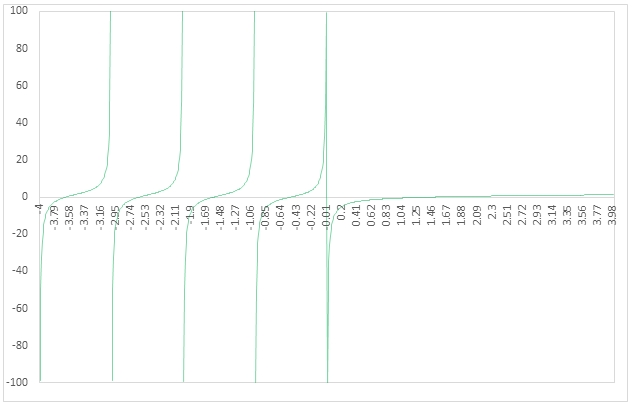# SQL Server digamma function

DIGAMMA
Updated: 20 January 2017
Use the SQL Server scalar function DIGAMMA to calculate digamma(z). Abramowitz and Stegun in Handbook of Mathematical Functions (6.3.1, p.258) defined the digamma function as:Syntax
SELECT [wct].[DIGAMMA](
<@X, float,>)
Arguments

 Input Name Definition @X The value of interest

Return Type
float
Remarks
·         When @X is a negative integer NULL is returned.
·         When @X = 0 zero is returned
·         Available in XLeratorDB / statistics 2008 only
Examples
Example #1
In the example we evaluate the function at x = 5.
SELECT wct.DIGAMMA(5) as psi
This produces the following result.
Example #2
In this example we use the SeriesFloat function from the XLeratorDB math library to generate a series on numbers from -4 to 4 in steps of .01, calculate the psi value for each number in the series, paste the results to Excel and create a simple graph of the function for the range.
SELECT
SeriesValue as z
,wct.Digamma(SeriesValue) psi
FROM
wct.SeriesFloat(-4,4,.01,nULL,NULL)
This produces the following result.### SupportCopyright 2008-2023 Westclintech LLC         Privacy Policy        Terms of Service